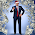## Thursday, June 18, 2015

### Unified Physics Snatches Pebble from Mainstream Master

The proton to electron mass ratio: $$\mu={m_p\over m_e}= {\alpha^2\over \pi r_pR_H}=1836.15267\;\;\leftarrow\;significant!!!$$
& the Planck mass to electron mass ratio:
$${m_{\ell}\over m_e}={\alpha^2\over{4\pi\ell R_H}}=2.3893048e+22$$
using $$m_pr_p=4\ell m_{\ell}=r_em_e\;where\;r_e={\alpha^2\over\pi R_H}$$

$\alpha=$ fine-structure constant
$r_p=$ proton radius
$R_H=$ Rydberg constant
$r_e={\alpha^2\over\pi R_H}=$ will define this term later (effective radius??? atomic radius?)
$\ell=$ Planck $\ell$ength
$\pi=3.1415926535897932384626433832795028841971693993751058209749445923078164\dots$

This is significant proof that Nassim Haramein's proton radius solution IS correct as it works in harmony with mainstream solutions to solve for natural dimensionless constants that have never before been analytically determined (except for the Rydberg constant, $R_H$).
(see previous post on proton-electron mass ratio and Planck mass to electron mass ratio for Google calculator links for ratio calculations)
The Surfer, OM-IV

1.The Planck to electron mass ratio is possibly a composite type number, that made of factors of 2 and a few or 2 primes.... there is some significance to investigating the details of this "constant" or large number ratio or inverse thereof... in other words, there is still much ongoing research... (if it's 2 primes, then there is more evidence of the dual-toroidal nature of the proton)

2.there will be a minor update soon - there a minor typo - inversion in an equation... this math is simple enough, so it'll be corrected in a bit...

3.there will be a minor update soon - there a minor typo - inversion in an equation... this math is simple enough, so it'll be corrected in a bit...

4.Ok, updated the Planck Mass to Electron Mass ratio. had an inversion....added where Lyz showed how the electron balances the proton.

5.I did this work in a rush while working a full time job, presenting in Scottsdale, and buying a new house, and taking care of all the stuff that comes up in life. Imagine what I could do if I was working on this full time. So, we will simply continue solving all of the remaining unsolved physics problems, step by step, one by one...

6.Me = mass of electron

7.updated ratios due to numerical resolution of my original calculations... fix is rippled through blog posts.... -mr

Watch the water. 🦆